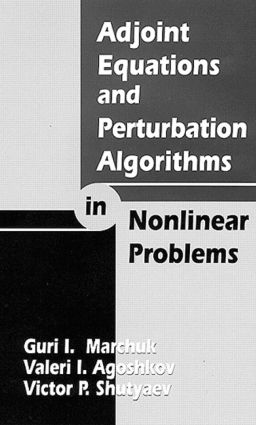Adjoint Equations and Perturbation Algorithms in Nonlinear Problems

1st Edition

CRC Press

288 pages

Hardback: 9780849328718
pub: 1996-05-07
\$145.00
x
eBook (VitalSource) : 9781315136707
pub: 2018-04-24
from \$72.50

FREE Standard Shipping!

Description

Sparked by demands inherent to the mathematical study of pollution, intensive industry, global warming, and the biosphere, Adjoint Equations and Perturbation Algorithms in Nonlinear Problems is the first book ever to systematically present the theory of adjoint equations for nonlinear problems, as well as their application to perturbation algorithms. This new approach facilitates analysis of observational data, the application of adjoint equations to retrospective study of processes governed by imitation models, and the study of computer models themselves. Specifically, the book discusses:

• Principles for constructing adjoint operators in nonlinear problems

• Perturbation algorithms using the adjoint equations theory for nonlinear problems in transport theory, quasilinear motion, substance transfer, and nonlinear data assimilation

• Known results on adjoint equations and perturbation algorithms in nonlinear problems

This groundbreaking text contains some results that have no analogs in the scientific literature, opening unbounded possibilities in construction and application of adjoint equations to nonlinear problems of mathematical physics.

Principles of Construction of Adjoint Operators in Non-Linear Problems

Construction of Adjoint Operators Based on Using the Lagrange Identity

Definition of Adjoint Operators Based on Using Taylor's Formula

Operators of the Class D and their Adjoint Operators

Properties of Adjoint Operators Constructed on the Basis of Various Principles

General Properties of Main and Adjoint Operators Corresponding to Non-Linear Operators

Properties of Operators of the Class D

Properties of Adjoint Operators Constructed with the Use of the Taylor Formula

Solvability of Main and Adjoint Equations in Non-Linear Problems

Solvability of the Equation F(u) = y

Solvability of the Equation A(u)v = y

Solvability of the Equation Ã(u)v = y

Solvability of the Equation A*(u)w = p

Solvability of the Equation Ã*(u)w = p

Transformation Groups, Conservation Laws and Construction of the Adjoint Operators in Non-Linear Problems

Definitions. Non-Linear Equations and Operators. Conservation Laws

Transformation of Equations

General Remarks on Constructing the Adjoint Equations with the Use of the Lie Groups and Conservation Laws

Construction of Adjoint Operators with Prescribed Properties

The Noether Theorem, Conservation Laws and Adjoint Operators

On Some Applications of Adjoint Equations

Perturbation Algorithms in Non-Linear Problems

Perturbation Algorithms for Original Non-Linear Equations and Equations Involving Adjoint Operators

Perturbation Algorithms for Non-Linear Functionals Based on Using Main and Adjoint Equations

Spectral Method in Perturbation Algorithms

Justification of the N-th Order Perturbation Algorithms

Convergence Rate Estimates for Perturbation Algorithms. Comparison with the Successive Approximation Method

Justification of Perturbation Algorithms in Quasi-Linear Elliptic Problems

Adjoint Equations and the N-th Order Perturbation Algorithms in Non-Linear Problems of Transport Theory

Some Problems of Transport Theory

The N-th Order Perturbation Algorithms for an Eigenvalue Problem

A Problem of Control and its Approximate Solution with the Use of Perturbation Algorithms

Investigation and Approximate Solution of a Non-Linear Problem for the Transport Equation

Adjoint Equations and Perturbation Algorithms for a Quasilinear Equation of Motion

Statement of the Problem. Basic Assumptions. Operator Formulation

Transformation of the Problem. Properties of the Non-Linear Operator

An Algorithm for Computing the Functional

The Problem on Chemical Exchange Processes

Adjoint Equations and Perturbation Algorithms for a Non-Linear Mathematical Model of Mass Transfer in Soil

Mathematical Models of Mass Transfer in Soil

Formulation of a Non-Linear Mathematical Model

Transformation of the Problem. Properties of the Non-Linear Operator

Approximate Solution of the Problem on Finding an Effective Dispersion Coefficient

An Algorithm for Solving the Problem

Applications of Adjoint Equations in Science and Technology

Adjoint Equations in Data Assimilation Problems

Application of Adjoint Equations for Solving the Problem of Liquid Boundary Conditions in Hydrodynamics

Shape Optimization Using Adjoint Equation Approaches

Global Transport of Pollutants

Problems of Climate Change Sensitivity in Various Regions of the World